# Lines and angles class 9 extra questions

Given below are the Lines and angles class 9 extra questions and important questions
• Concepts questions
• Calculation problems
• Multiple choice questions
• Fill in the blanks

Question 1
Write the type of angles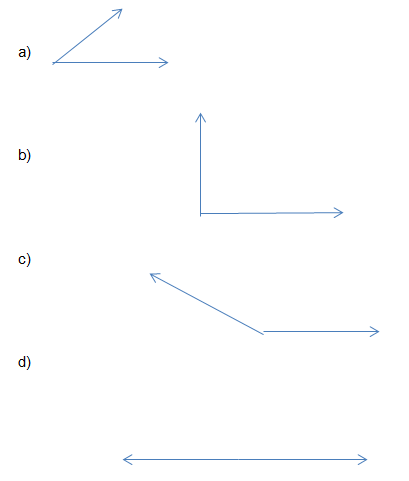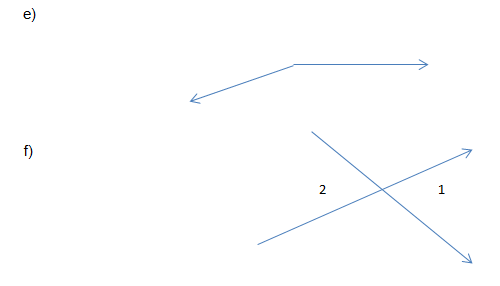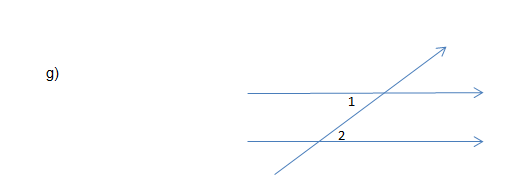Solution
a. Acute angle
b. Right angle
c. Obtuse angle
d. Straight angle
e. Reflex angle
f. Vertically opposite angle
g. Alternate interior angles

Question 2
True or False statement
a. Pairs of vertically opposite angles is always equal
b. The sum of the angles of a triangle is $180^0$
c. If the sum of two adjacent angles is $45^0$, then two adjacent angles are acute angles
d. If a line is perpendicular is one of two parallel lines, then it is also perpendicular to the other
e. Two lines are intersected by the transversal, and then the corresponding angles are equal
f. Can we have a triangle where all the interior angles are more than $60^0$
g. Sum of two complimentary angles is equal to $90^0$
h. Sum of all the exterior angles of any polygon is always $360^0$
Solution
• True
• True
• True
• True
• False
• False
• True
• True

## Multiple choice Questions

Question 3
Find the value of x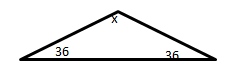a. $98^0$
b. $100^0$
c. $108^0$
d. $96^0$
Solution
(c)
$x+36+36=180$ => $x=108^0$

Question 4
A pair of angles is called linear pair if the sum of two adjacent angles is?
a.$180^0$
b.$90^0$
c.$270^0$
d.$360^0$
Solution
(a)

Question 5
Find the value of x,y and z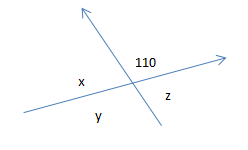a. $x=110^0,y=70^0,z=80^0$
b. $x=70^0,y=110^0,z=60^0$
c. $x=70^0,y=100^0,z=70^0$
d. $x=70^0,y=110^0,z=70^0$
Solution
(d)
Vertically opposite angle theorem and linear pair axiom can be used to find the answer

Question 6
An exterior angle of the triangle is $110^0$. And its two opposite interior angles are in the ratio 5:6. What are the values of those angles?
a. $50^0,60^0$
b. $25^0,30^0$
c. $35^0,42^0$
d. $40^0,48^0$
Solution
(a)

Question 7
Lines l || k and m || n .Find the value of angle z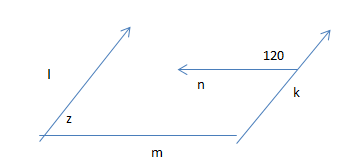a. $45^0$
b. $60^0$
c. $70^0$
d. $50^0$
Solution
(b)

Question 8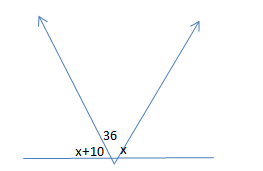Find the value of x
a. $67^0$
b. $71^0$
c. $57^0$
d. None of these
Solution
(a)

Question 9
The side BC, AB and AC of the triangle ABC are produced in order forming exterior angles $\angle ACD =x$ , $\angle BAE =y$ and $\angle CBF=z$ then the value of $2x +2y + 2z$ is
a. 180°
b. 360°
c. 540°
d. 720°
Solution
(d)
Let a , b,c be the angles of triangle, then a+b + c=180
Now x=a+b, y=b+c, z=a+c
Therefore 2x + 2y + 2z=4(a+ b+c)=720

Question 10
The angles of the triangles are in the ration 1:2:3. then the triangle is a. scalene
b. obtuse angled
c. acute angled
d. right angles
Solution
(d)
Let x , 2x,3x be the angles of triangle, then x+2x + 3x=180
Therefore x=30
So angles are 30,60 anmd 90

## Fill in the blanks

Question 11
a.Sum of two supplementary angles is ______
b. Two lines parallel to the same line is ____ each other
c. An acute angle is always less than _____
d. Angles forming a linear pair are ______
e. If one angle of triangle is equal to the sum of other two angles, then the triangle is ______
f. if two straight lines intersect ,the adjacent angles are ______
Solutions
a. $180^0$
b. parallel
c. $90^0$
d. supplementary
e. right angle triangle
f. Supplementary

## Table Type Question

Question 12
 Angle $30^0$ $80^0$ $11^0$ $87^0$ Complementary angle Supplementary angle
Solution:
Complementary =$90- x$
Supplementary =$180 -x$

Question 13
In the below figure, DE || QR and AP and BP are bisectors of $\angle EAB$ and $\angle RBA$, respectively. Find $\angle APB$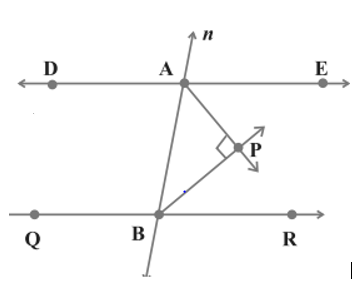Solutions
As DE || QR , we have $\angle EAB + \angle RBA =180$
Now in Triangle APB
$\angle APB + \angle BAP + \angle PBA =180$
Now as AP and BP are bisectors
$\angle APB + \frac {1}{2} \angle EAB + \frac {1}{2} \angle RBA=180$
$\angle APB + \frac {1}{2} ( \angle EAB + \angle RBA )=180$
$\angle APB + 90=180$
$\angle APB =90$

Question 14
In the below figure AB || CD, find the value of angle y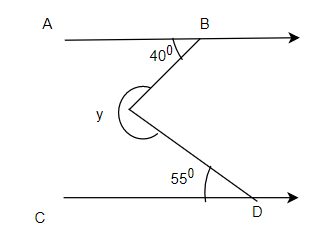Solutions
we can draw a parallel line with AB through the point of intersection as shown below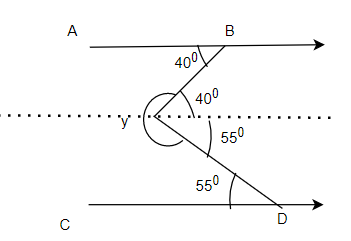Then angle y will be
$y= 360- 40-55=265$ °

Question 15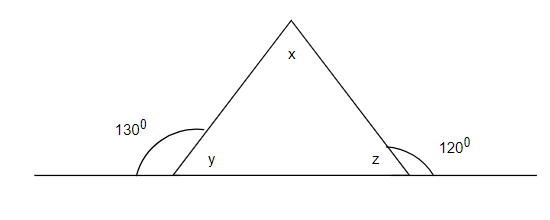find the values of x,y and z
Solutions
y=180-130=50°
z=180-120=60°
Now x= 180-y-z=70°

Question 16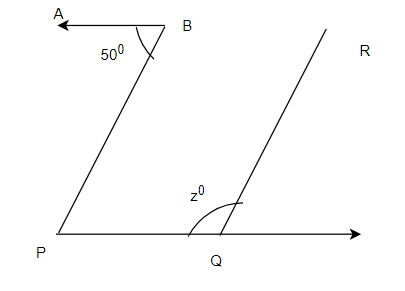In the above figure, AB ||PQ and PB||QR, find the value of angle z
Solutions
Since AB ||PQ , $\angle PBQ =50$°
Now since PB||QR, we have
z+50=180
z=130°

## Summary

This Lines and angles class 9 extra questions with solutions is prepared keeping in mind the latest syllabus of CBSE . This has been designed in a way to improve the academic performance of the students. If you find mistakes , please do provide the feedback on the mail.